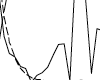# Impact of the Form of the Momentum Equation on Shallow Water Models based on the Generalized Wave Continuity EquationA number of finite-element based shallow water models use the GWC (generalized wave continuity) algorithm, first introduced by Lynch and Gray in 1979, to suppress short wave noise without artificial or numerical damping. Nearly all of these, including the ADCIRC (ADvanced 3D CIRCulation model developed by Westerink and Luettich) model used in our research, utilize the non-conservative form of the momentum equation to obtain the velocity field. However, as early as 1990, it was discovered that the inconsistent treatment of the advective terms between the GWC equation (which uses the conservative form of the momentum equation in its formulation) and the non-conservative momentum (NCM) equation was causing instabilities. Additional analyses by Kinnmark and Aldama, among others, provided further evidence that the conservative form of the momentum (CM) equation may improve accuracy and stability, particularly for simulations that have regions of highly advective flows, e.g., converging flow near inlets and around barrier islands. Furthermore, as the ADCIRC modeling group begins to explore alternative algorithms for the continuity equations (e.g., discontinuous Galerkin algorithm instead of GWC), the conservative form of the momentum equation would facilitate model coupling. For these reasons, we modified the ADCIRC code to use the conservative form of the momentum equation. The impact of this change on mass balance, stability, and accuracy (spatial and temporal) is rigorously assessed, first for ID barotropic flows and later for 2D barotropic flows in a variety of basins. Results from the 1D experiments indicate the following: global mass balance improves up to two orders of magnitude, depending on the domain; local mass balance greatly improves in areas where steep bathymetry gradients occur; when combined with a predictor-corrector time-marching algorithm, stability increases by at least a factor of two (for a given level of mass balance error); and the form of the momentum equation does not cause a significant change in temporal or spatial accuracy when evaluating the two different time-marching algorithms.